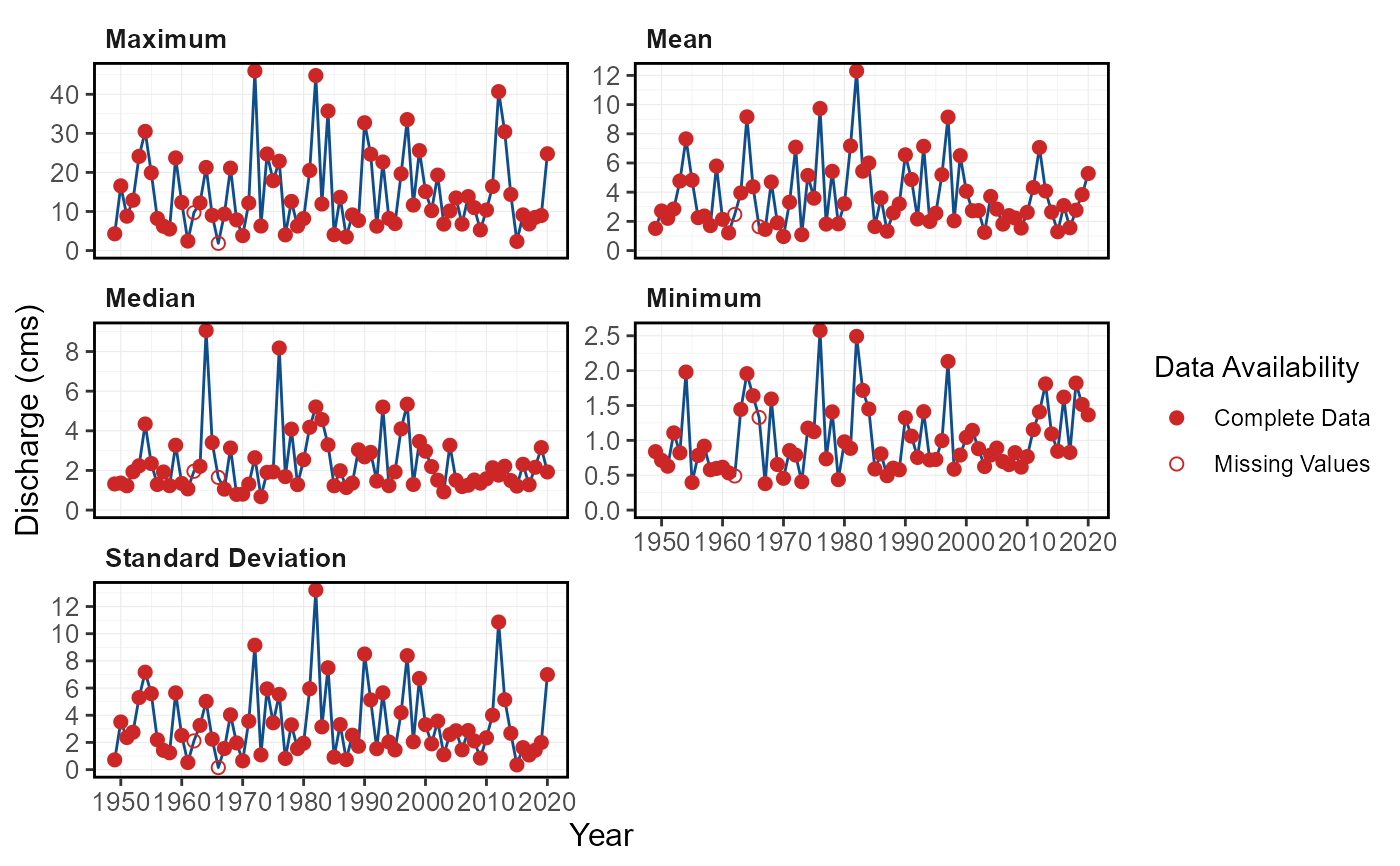Plots the mean, median, maximum, minimum, standard deviation of annual flows. Calculates statistics from all values, unless specified. Data calculated using screen_flow_data() function. Returns a list of plots.

plot_data_screening(
data,
dates = Date,
values = Value,
groups = STATION_NUMBER,
station_number,
roll_days = 1,
roll_align = "right",
water_year_start = 1,
months = 1:12,
start_year,
end_year,
include_title = FALSE
)

## Arguments

data Data frame of daily data that contains columns of dates, flow values, and (optional) groups (e.g. station numbers). Leave blank if using station_number argument. Name of column in data that contains dates formatted YYYY-MM-DD. Only required if dates column name is not 'Date' (default). Leave blank if using station_number argument. Name of column in data that contains numeric flow values, in units of cubic metres per second. Only required if values column name is not 'Value' (default). Leave blank if using station_number argument. Name of column in data that contains unique identifiers for different data sets, if applicable. Only required if groups column name is not 'STATION_NUMBER'. Function will automatically group by a column named 'STATION_NUMBER' if present. Remove the 'STATION_NUMBER' column beforehand to remove this grouping. Leave blank if using station_number argument. Character string vector of seven digit Water Survey of Canada station numbers (e.g. "08NM116") of which to extract daily streamflow data from a HYDAT database. Requires tidyhydat package and a HYDAT database. Leave blank if using data argument. Numeric value of the number of days to apply a rolling mean. Default 1. Character string identifying the direction of the rolling mean from the specified date, either by the first ('left'), last ('right'), or middle ('center') day of the rolling n-day group of observations. Default 'right'. Numeric value indicating the month (1 through 12) of the start of water year for analysis. Default 1. Numeric vector of months to include in analysis (e.g. 6:8 for Jun-Aug). Leave blank to summarize all months (default 1:12). Numeric value of the first year to consider for analysis. Leave blank to use the first year of the source data. Numeric value of the last year to consider for analysis. Leave blank to use the last year of the source data. Logical value to indicate adding the group/station number to the plot, if provided. Default FALSE.

## Value

A list of ggplot2 objects with the following for each station provided:

Data_Screening

a plot that contains annual summary statistics for screening

Default plots on each object:
Minimum

annual minimum of all daily flows for a given year

Maximum

annual maximum of all daily flows for a given year

Mean

annual mean of all daily flows for a given year

StandardDeviation

annual 1 standard deviation of all daily flows for a given year

## See also

screen_flow_data

## Examples

# Run if HYDAT database has been downloaded (using tidyhydat::download_hydat())
if (file.exists(tidyhydat::hy_downloaded_db())) {

# Plot screening statistics using a data frame and data argument with defaults
flow_data <- tidyhydat::hy_daily_flows(station_number = "08NM116")
plot_data_screening(data = flow_data)

# Plot screening statistics using station_number argument with defaults
plot_data_screening(station_number = "08NM116")

# Plot screening statistics for water years starting in October
plot_data_screening(station_number = "08NM116",
water_year_start = 10)

# Plot screening statistics for 7-day flows for July-September months only
plot_data_screening(station_number = "08NM116",
roll_days = 7,
months = 7:9)

}
#> \$Data_Screening#>